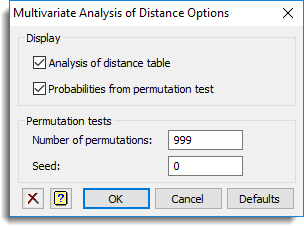1. Home
2. Multivariate Analysis of Distance Options

# Multivariate Analysis of Distance Options

Specifies what output should be produced by the multivariate analysis of distance.## Display

Specifies which items from the multivariate analysis of distance are to be displayed in the Output window.

 Analysis of distance table Displays an analysis-of-distance table giving the sums of squared distances between data points for each model term. Probabilities from permutation test Adds a column to the analysis-of-distance table containing probabilities from the permutation test for each model term.

## Permutation tests

Specify options for the permutation tests.

 Number of permutations This options specifies the number of permutations used in calculating the probabilities, default 999. Seed Specifies the seed for the random number generator used to make the permutations; default 0 continues from the previous generation or (if none) initializes the seed automatically.

## Action buttons

 OK Save the option settings and close the dialog. Cancel Close the dialog without making any changes. Defaults Reset the options to their default settings.

## Action IconsClear Clear all fields and list boxes.Help Open the Help topic for this dialog.